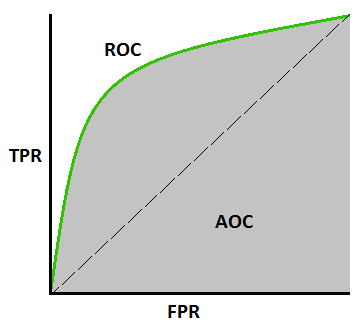# ROC曲线与AUC值

### 混淆矩阵 - confusion matrix

 True condition Total population Condition positive Condition negative Predictedcondition Predicted conditionpositive True positive (TP)Power False positive (FP)Type I error Predicted conditionnegative False negative (FN)Type II error True negative (TN)

• 真阳性率(True positive rate): $$TPR = \frac{TP}{Condition\,positive} =\frac{TP}{TP + FN}$$
• 假阳性率(False positive rate): $$FPR = \frac{FP}{Condition\,negative} = \frac{FP}{FP + TN}$$
• 真阴性率(True negative rate): $$TNR = \frac{TN}{Condition\,negative} = \frac{TN}{FP + TN}$$
• 假阴性率(False negative rate): $$FNR= \frac{FN}{Condition\,positive}=\frac{FN}{TP + FN}$$

### ROC曲线

ROC曲线用于衡量二分类模型的性能，横坐标表示假阳性率(FPR)，纵坐标表示真阳性率(TPR)。显然ROC的横纵坐标都在$$[0,1]$$之间。ROC Curve